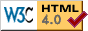```
```
EXAMPLE

Particulars of a pin-ended column made from a 250 MPa yield steel are :
L = 1 m       A = 103 mm2       I = 105 mm4       η = 0.3
What load can it support if a safety factor of 2 is specified ?

Properties :
r = √(I/A) = 10 mm       ρ = L/r = 100       σc = E(π/ρ)2 = 204 MPa
From ( 2) with   S = 250 MPa, the direct stress at failure is   σd = 134 MPa   ( note that   σd must be less than both σc and S ).
The failure load is therefore this mean stress times the area,   A, ie.   134 kN.
So if the safety factor is 2, the permissible load will be   134/2 = 67 kN.

Let us see what would happen if this had been carried out   incorrectly with safety factor applied to strength rather than to load.
From ( 2) with   S = Sy /n = 250/2 = 125 MPa, the direct stress is found to be   83 MPa. Multiplying by the area, the corresponding load is   83 kN. Hence the   incorrect conclusion is that the column could withstand a load of   83 kN with a safety factor of   2.
But if the   83 kN load were actually applied then the safety factor is   n = failure load/actual load = 134/83 = 1.6 - significantly less than the   2 expected.Copyright 1999-2005 Douglas Wright,   doug@mech.uwa.edu.au
last updated May 2005